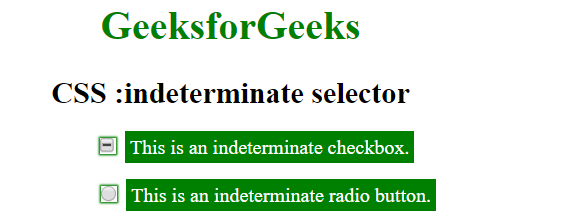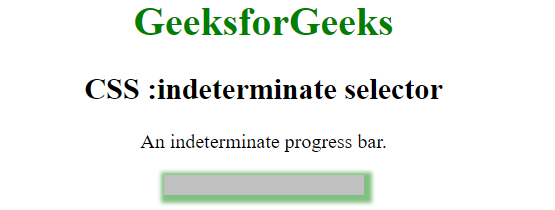# CSS :indeterminate Selector

The :indeterminate selector in CSS is used to select any form elements that are in indeterminate state i.e a state that is neither checked nor unchecked. Elements targeted by this selector are:

• <input = “checkbox”> elements whose indeterminate property is set to true by JavaScript
• <input = “radio”> elements, when all radio buttons with the same name value in the form are unchecked
• <progress> elements in an indeterminate state

Example 1:

## html

 `` `<``html``>`   `<``head``>` `    ``<``title``>` `        ``CSS :indeterminate selector` `    ``` `    ``<``style``>` `        ``input:indeterminate+label {` `            ``background: green;` `            ``color: white;` `            ``padding: 4px;` `        ``}`   `        ``input:indeterminate {` `            ``box-shadow: 0 0 1px 1px green;` `        ``}` `    ``` ``   `<``body` `style``=``"text-align: center;"``>` `    ``<``h1` `style``=``"color:green;"``>` `        ``GeeksforGeeks` `    ``` `    ``<``h2``>` `        ``CSS :indeterminate selector` `    ``` `    ``<``div``>` `        ``<``input` `type``=``"checkbox"` `id``=``"checkbox"``>` `        ``<``label` `for``=``"checkbox"``>` `            ``This is an indeterminate checkbox.` `        ``` `    ``` `    ``<``br``>` `    ``<``div``>` `        ``<``input` `type``=``"radio"` `id``=``"radio"` `name``=``"abc"``>` `        ``<``label` `for``=``"radio"``>` `            ``This is an indeterminate radio button.` `        ``` `    ```   `    ``<``script``>` `        ``let doc = document.getElementsByTagName("input");`   `        ``for (let i = 0; i < ``doc.length``; i++) {` `            ``doc[i]``.indeterminate` `= ``true``;` `        ``}` `    ``` ``   ``

Output:Example 2:

## html

 `` `<``html``>`   `<``head``>` `    ``<``title``>` `        ``CSS :indeterminate selector` `    ``` `    ``<``style``>` `        ``progress:indeterminate {` `            ``opacity: 0.5;` `            ``background: lightgray;` `            ``box-shadow: 2px 2px 4px 4px green;` `        ``}` `    ``` ``   `<``body` `style``=``"text-align: center;"``>` `    ``<``h1` `style``=``"color:green;"``>` `        ``GeeksforGeeks` `    ``` `    ``<``h2``>` `        ``CSS :indeterminate selector` `    ``` `    ``<``p``>An indeterminate progress bar.` `    `  ``   ``

Output:Supported Browsers: The browser supported by :indeterminate selector are listed below:

• Apple Safari 3.0